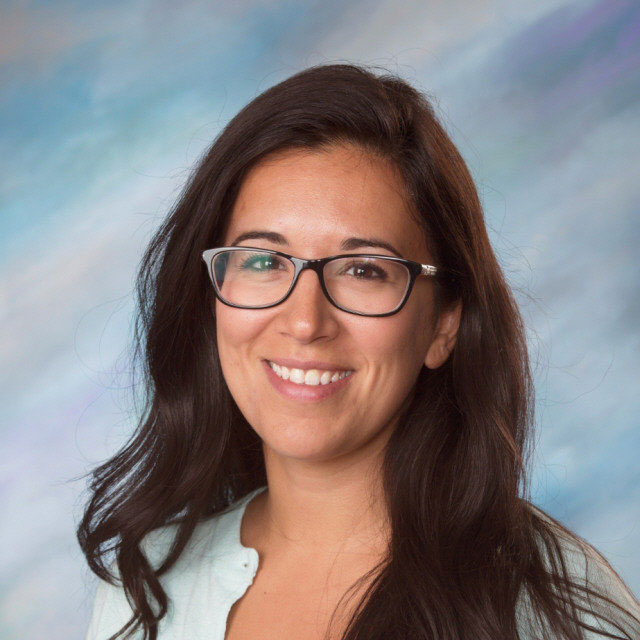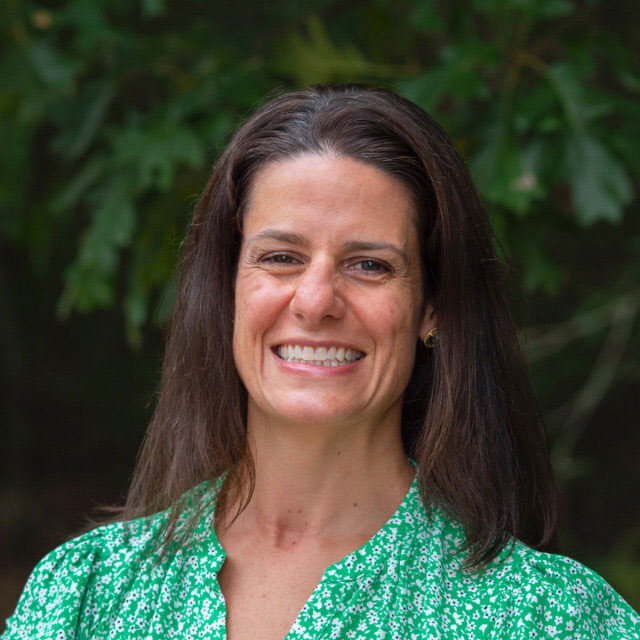# Mathematics

Honors, and the College Prep Math courses are offered for students in grades 9, 10, 11, & 12. The Honors courses are designed for those students who have a strong background in mathematics and are planning on pursuing higher education. College Prep courses are designed for students planning on attending technical schools, two-year colleges or furthering their study in their chosen vocational area, entering the workforce or military service. All levels prepare students in their freshman and sophomore years for the MCAS exam. In their junior year, the course work becomes more specific to graduation plans. Placement in all mathematics courses after Algebra I is contingent upon successful completion of the prerequisites and teacher recommendation. All students must pass four years of mathematics.

## Courses

This course covers the traditional topics in algebra focusing on fundamental properties of real numbers, linear equations, absolute value, inequalities, factoring, quadratic equations, data analysis and problem solving.

This course in algebra gives students a strong foundation of algebraic skills. Topics include fundamental properties of real numbers, linear equations, absolute value, inequalities, factoring, quadratic equations, and data analysis. Modeling and problem solving skills are stressed. It is recommended for students who are capable of doing work on an advanced level and at an accelerated pace.

Freshmen who have shown proficiency of the Algebra I standards on the math placement test will be enrolled in this class. This course is recommended for students with a strong mathematical background capable of doing advanced work on a daily basis. Topics covered include an in-depth study of linear and quadratic equations, inequalities, systems of equations, graphing, polynomial functions, and factoring, operations with rational expressions, polynomial division and synthetic division, powers, roots, radicals, exponential and logarithmic functions, polynomial functions, rational functions, and conic sections.

This course is recommended for those students who are capable of doing challenging work on a regular basis. Topics include plane geometry, formal proofs, coordinate geometry, area, perimeter, volume and other properties of polygons, parallels, triangle congruence, circles, and special right triangles including Pythagorean Theorem.

This course covers properties of angles, parallel lines, triangles, geometric proofs, circles, area, perimeter and volume of polygons, congruence and similarity of triangles, special right triangles and Pythagorean Theorem.

This course is recommended for students with strong mathematical ability who are planning to further their education at a four-year college. It reviews algebra topics necessary for success in calculus and further study of coordinate geometry, polynomial functions of higher degree, synthetic division, complex numbers, and the fundamental theorem of algebra, and parent functions. Right triangle trigonometry, general angles and radian measures, inverse trigonometric functions, solving right triangles, laws of sines and cosines, graphs of trigonometric functions and their translations, and trigonometric identities are also covered.

This course is recommended for students with a strong mathematical background capable of doing advanced work on a daily basis. Topics covered include an in depth study of linear and quadratic equations, inequalities, systems of equations, graphing, polynomial functions, and factoring, operations with rational expressions, polynomial division and synthetic division, powers, roots, radicals, exponential and logarithmic functions, polynomial functions, rational functions, and conic sections.

This course provides a continuation of the study of algebra for those students planning on additional education after graduation. It reviews in depth topics from Algebra I such as properties of real numbers, linear and quadratic equations, inequalities, graphing functions, add, subtract, multiply and divide polynomials, factoring, operations with rational expressions, polynomial division and synthetic division.

AP Calculus AB is an introductory college-level calculus course, with a focus on differential and integral calculus. Student work is demonstrated in both verbal and written forms, and instructors focus not only content, but also the underlying mathematical concepts. Topics are considered analytically, numerically, graphically and verbally. Students in this course also prepare exclusively for the AP Calculus AB exam and often use materials created and recommended by the College Board. Students must successfully complete Pre-Calculus before taking AP Calculus AB.

This course is recommended for students with strong mathematical ability and reviews algebra topics necessary for success in calculus and further study of coordinate geometry, polynomial functions of higher degree, synthetic division, complex numbers, and the fundamental theorem of algebra, and parent functions. Right-triangular trigonometry, general angles and radian measures, inverse trigonometric functions, solving right triangles, laws of sines and cosines, graphs of trigonometric functions and their translations, and trigonometric identities are also covered.

Students will learn fundamental concepts of Trigonometry. Concepts will include defining trigonometric functions and angle. Right Triangle Trigonometry and its applications will be discussed throughout the year. Applying trigonometric function by using the inverse, cofunctions, evaluating functions and applications of functions along with applying radian and degree measure will be major focuses. Students will be exposed to graphs of functions and they will also be exposed to the basic Trigonometric Identities and its functions. In addition, students will solve triangles using the Law of Sines and Cosines.

The Algebra & Trigonometry Applications course is a course of rigor that incorporates Algebra II and fundamental mathematical concepts, including right triangular trigonometry and its applications. Problems-of-the-Day may include PSAT, SAT, & Accuplacer style questions. The course promotes creativity, innovation, critical thinking, problem solving, communication and collaboration.

## Mathematics Faculty## Marcie Gamelin

Math
x150 mgamelin## Beth Jupin

Math
x156 bjupin## Ryan Lewis

Math
x183 rlewis## Craig Lincoln

Math
x157 clincolnMath
x172 spepin

## Apply Now

Leave your options open. Apply today and make your decision in March. 8th grade students from Acushnet, Carver, Lakeville, Mattapoisett, and Rochester are invited to begin the application process at https://oldcolony.go2cte.com.